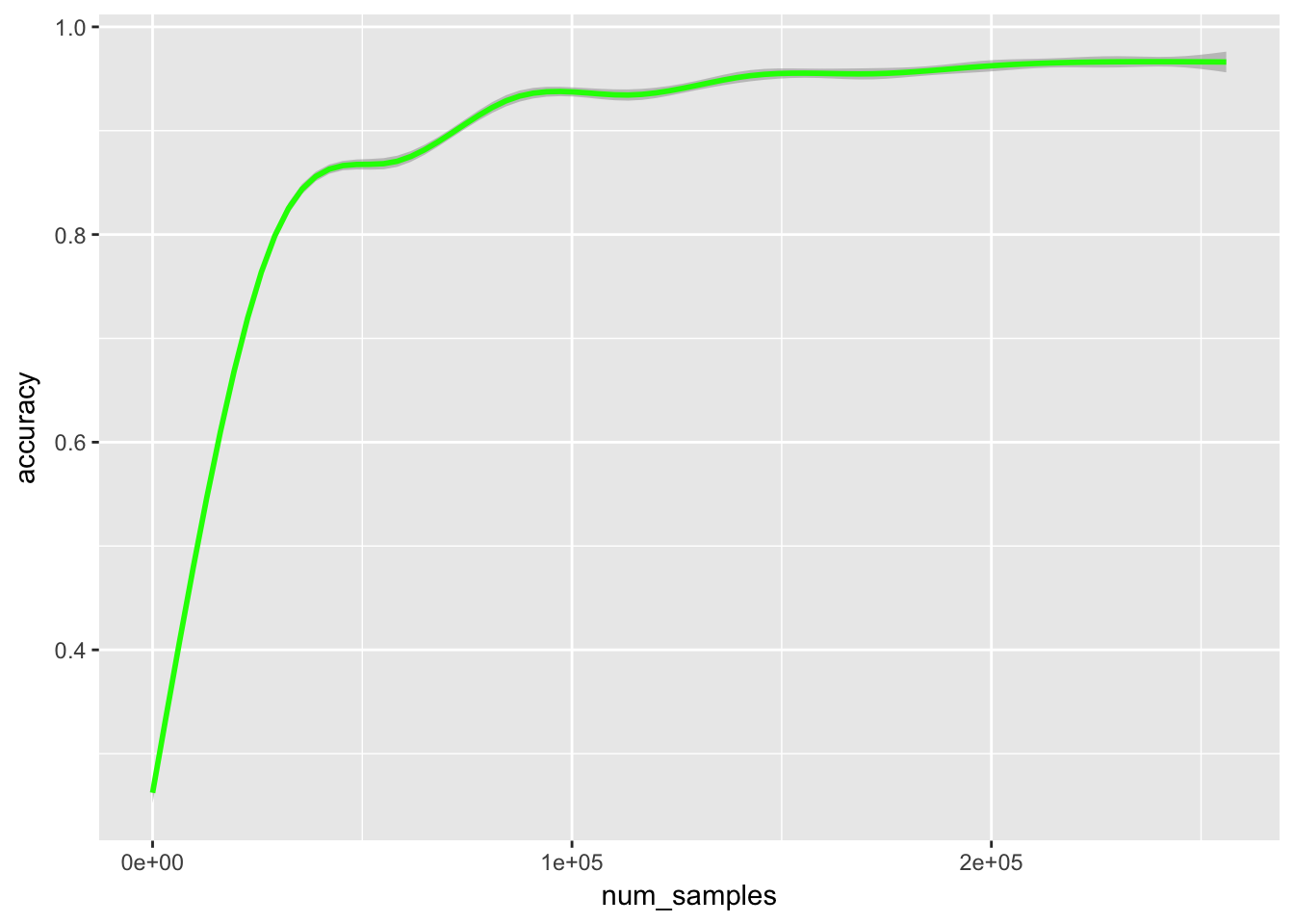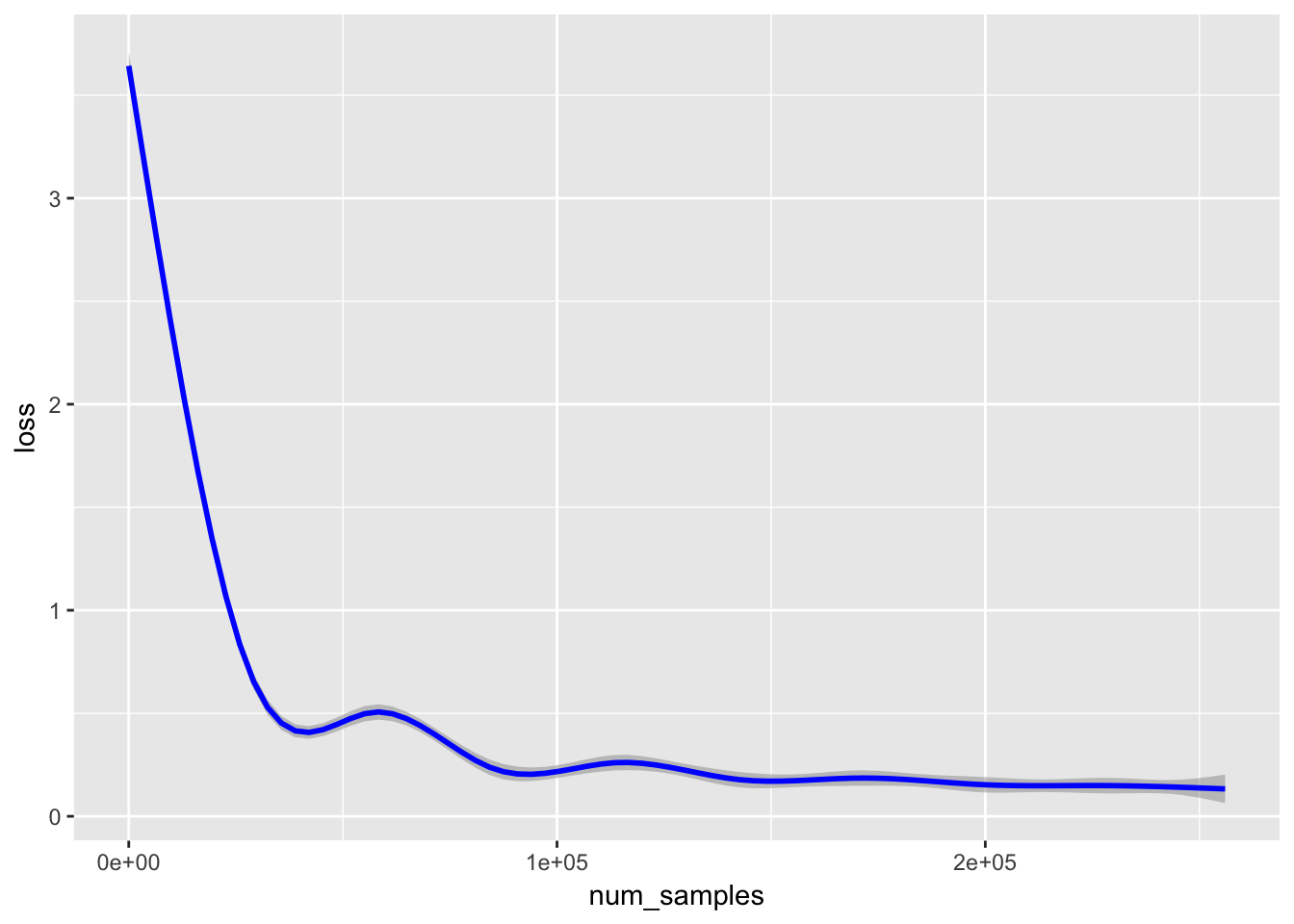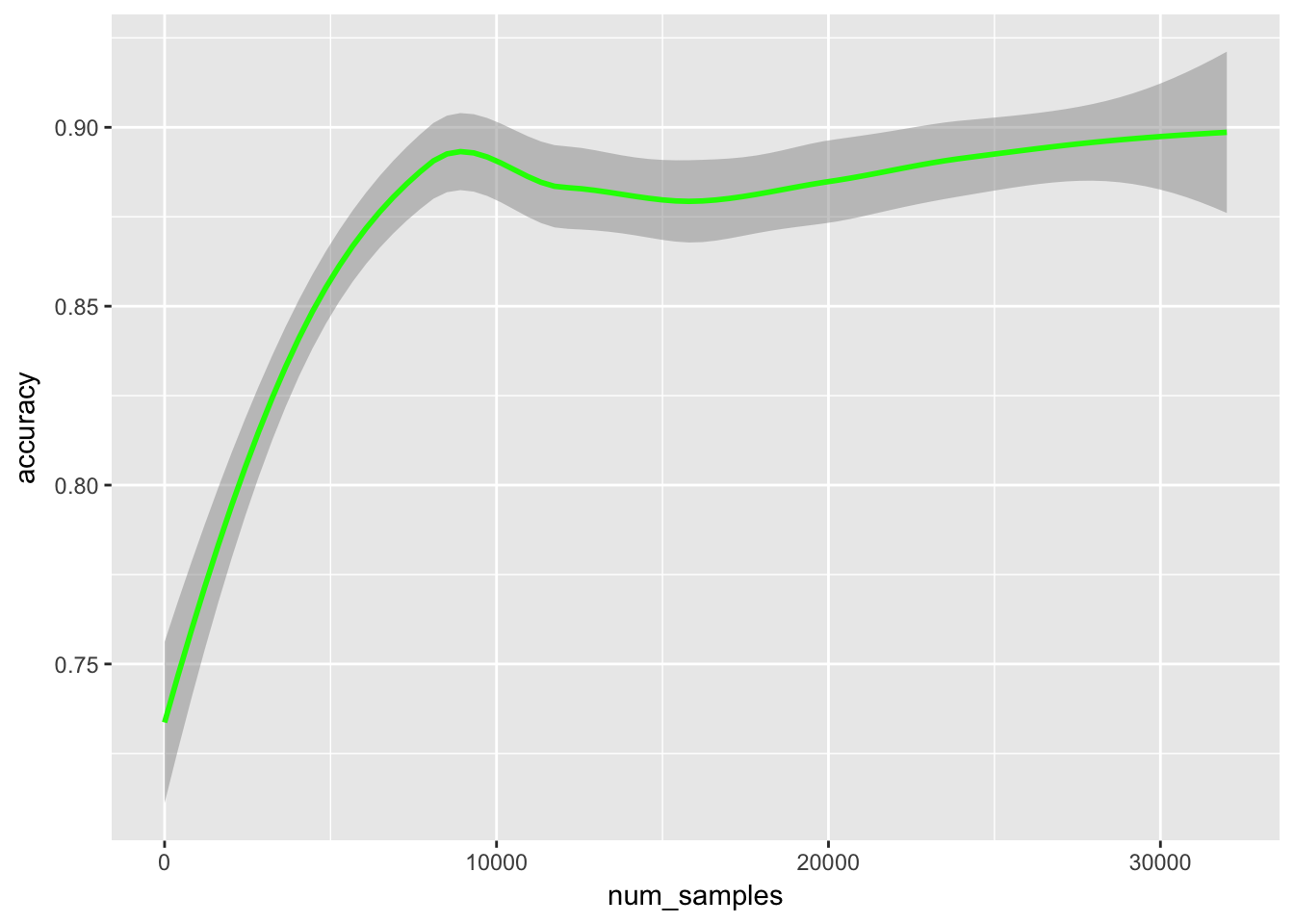## R Lab

Let’s build a simple logistic regression classifier from scratch.

Let’s first include our standard libraries.

``````# Tibbles!
library(tidyverse)
# Used for generating clusters
library(MASS)``````

For testing purposes let us include our data generator from Session 5:

``````generate_lda_clusters = function(classes, dim, num_samples) {
# generate a non-degenerate covariance matrix
repeat{
A = matrix(runif(dim^2),dim,dim)
cov_mat = A%*%t(A)
if(det(cov_mat)!=0)
break
}

# generate means
means = matrix(1.5*runif(dim*classes),dim,classes)

#initialize data frame
xvals = matrix(numeric(dim*classes*num_samples), num_samples*classes, dim)
y = matrix(numeric(classes*num_samples),classes*num_samples, 1)
t = as.tibble(cbind(xvals,y))
colnames(t)=c(paste0('x',1:dim),'y')

# Fill data frame
for(ix in 0:(num_samples*classes-1)) {
c = (ix %/% num_samples)+1
t[(ix+1),]=c(mvrnorm(1,means[,c],cov_mat),c)
}
# Generate output
output = list(data = t, means = t(means), covariance = cov_mat)
return(output)
}``````

Now I’m going to add some convenience functions:

``````bias = function(x) {
num_times = dim(x)
as.matrix(cbind(x,rep(1,num_times)))
}

OHE = function(x) {
#Get list of unique entries
values = unique(sort(x))
num_values = length(values)
encoder = function(z){
#find index
ix = match(z,values)
#initialize zero vector
v = numeric(num_values)
v[ix]=1
return(v)
}

#vectorized encoder
function(y) {
matrix(unlist(map(y,encoder)),length(y),num_values,byrow=T)

}
}

Clipper = function(x) { as.matrix(apply(x, c(1,2), function(z) max(min(z,exp(30)),exp(-30)))) }
Scaler = function(x) {
num_samples = dim(x)
num_features = dim(x)
means = numeric(num_features)
vars = numeric(num_features)

#calculate means

means = apply(x, 2, mean)
vars = apply(x, 2, var)

function(z) {
if(dim(z)!=num_features) stop("Attempt to transform data with the incorrect number of features")

num_to_transform = dim(z)
w = matrix(numeric(num_features*num_to_transform), num_to_transform, num_features)
for(col in seq(num_features)) {
for(row in seq(num_to_transform)) {
w[[row,col]] = (z[[row,col]]-means[[col]])/sqrt(vars[[col]])
}
}
return(w)
}
}``````

I’m going to try to implement this as an ultralightweight “object”, by which I just mean an environment. This loses some of the functionality of R6 classes, but is simpler syntactically.

``````LogisticClassifier = function(tr_data, tr_labels, prior_std_dev = 0.1) {
num_samples = dim(tr_data)

num_features = dim(tr_data)

num_classes = dim(tr_labels)
training_log = tibble(num_samples = c(0), accuracy = c(0), loss = c(0))

beta = matrix(rnorm(num_features*num_classes)*prior_std_dev, num_features, num_classes)

# Shuffle rows
shuffle = sample.int(num_samples)
tr_data = tr_data[shuffle, ]
tr_labels = tr_labels[shuffle, ]

train_counter = 0
probs = function(x) {
batch_size = dim(x)
prbs = Clipper(exp(x %*% beta))
for(row in seq(batch_size))
prbs[row,]=prbs[row,]/sum(prbs[row,])
return(as.matrix(prbs))
}

train_on_batch = function(batch_size = 128, learning_rate = 0.1) {
to_select = ((train_counter+seq(0,batch_size-1)) %% num_samples)+1

data = as.matrix(tr_data[to_select,])
labels = as.matrix(tr_labels[to_select,])
multiplicand = probs(data)
beta <<- beta + (t(data)%*%(labels-multiplicand))*learning_rate/batch_size
train_counter <<- train_counter+batch_size
acc_counter = 0
error = 0
for(i in seq(batch_size)) {
if(which.max(multiplicand[i,])==which.max(labels[i,])) {
acc_counter = acc_counter+1
}
error=error-log(sum(multiplicand[i,]*labels[i,]))
}
acc_counter = acc_counter/batch_size
error = error/batch_size

training_log <<- add_row(training_log, num_samples = train_counter, accuracy = acc_counter,loss = error)
}
environment()
}``````

Test it out. Generate our data:

``````df = generate_lda_clusters(10,50,1000)
xvals = df\$data[,1:50]
yvals = df\$data[,51]
shuffle = sample.int(nrow(xvals))``````

See how we do:

``````sc = Scaler(xvals)
tc = bias(sc(xvals))
ohe = OHE(yvals\$y)
ty = ohe(yvals\$y)
x_train = tc[shuffle[1:9000],]
y_train = ty[shuffle[1:9000],]
x_test = tc[shuffle[9001:10000],]
y_test = ty[shuffle[9001:10000],]
logc = LogisticClassifier(x_train, y_train)``````
``````for(i in 1:4000) {
logc\$train_on_batch(batch_size = 64,learning_rate = 1.0)
}``````

Test this on our test data.

``````preds = logc\$probs(x_test)
acc = 0
for(i in seq(nrow(preds)))
if(which.max(preds[i,])==which.max(y_test[i,]))
acc = acc+1/nrow(preds)
print(acc)``````
``##  0.968``
``````library(ggplot2)
ggplot(data=logc\$training_log) +
geom_smooth(aes(x=num_samples, y=accuracy), color = "Green", alpha = 0.5) ````````````ggplot(data=logc\$training_log) +
geom_smooth(aes(x=num_samples, y=loss), color="Blue", alpha = 0.5)``````Okay onto the digits:

``````library(keras)
mnist = dataset_mnist()
to_sample = 1:5000
x_train = mnist\$train\$x
x_train = array_reshape(x_train, c(nrow(x_train),784))
pca = prcomp(x_train[to_sample,], rank.=50)
xvals = predict(pca, x_train)
sc = Scaler(xvals)
tc = bias(sc(xvals))
ohe = OHE(as.matrix(mnist\$train\$y))
ty = ohe(mnist\$train\$y)
mnistc = LogisticClassifier(tc, ty)
for(i in 1:500) {
mnistc\$train_on_batch(batch_size = 64, learning_rate = 1.0)
}``````

Test this on our test data.

``````to_test = 1:10000
x_test = mnist\$test\$x
x_test = array_reshape(x_test, c(nrow(x_test),784))
ttt = predict(pca, x_test[to_test,])
preds = mnistc\$probs(bias(sc(ttt)))
acc = 0
y_test = ohe(mnist\$test\$y)
for(i in seq(nrow(preds)))
if(which.max(preds[i,])==which.max(y_test[i,]))
acc = acc+1
print(acc/nrow(preds))``````
``##  0.8995``
``````ggplot(data=mnistc\$training_log) +
geom_smooth(aes(x=num_samples, y=accuracy), color = "Green", alpha = 0.5) ````````````ggplot(data=mnistc\$training_log) +
geom_smooth(aes(x=num_samples, y=loss), color = "Blue", alpha = 0.5) ``````